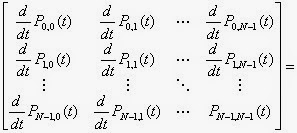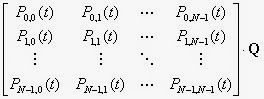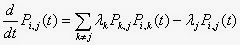## Friday, January 23, 2015

### Approximating a Continuous Time Markov ProcessFigure 1: Rate of Transitions Between States in a Three-State Markov Chain
1.0 Introduction

This post, about Markov processes, does not have much to do with economics. I here define how to approximate a continuous time Markov chain with a discrete time Markov chain. This mathematics is useful for one way of implementing computer simulations involving Markov chains. That is, I want to consider how to start with a continuous time model and synthesize a realization with a small, constant time step.

2.0 Continuous Time Markov Chains

Consider a stochastic process that, at any non-negative time t is in one of N states. Assume this process satisfies the Markov process: the future history of the process after time t depends only on the state of the process at time t, independently of how the process arrived at that state. I consider here only processes with stationary probability distributions for state transitions and for times between transitions. A continuous time Markov chain is specified by a state transition matrix. In this section, I define such a matrix as well as specifying two additional assumptions.

Formally, let Pi, J denote the conditional probability that the next transition will be into state j, given that the process is in state i at time zero. (As seen below, in the notation adopted here it matters that these conditional probabilities are not a function of time.) Assume that for each state, the next transition when the process is in that state is into a different state:

Pi, i = 0; i = 0, 1, ..., N - 1

Further, assume that for each state, the time to the next transition is from an exponential distribution with the rate of occurrence of state transitions dependent only on the initial state:

Fi, j(t) = 1 - e- λi t; i, j = 0, 1, ..., N - 1;

where Fi, j(t) is the conditional probability that the next transition will be before time t, given that the chain is in state i at time zero and that the next transition will be into state j. In other words, Fi, j(t) is the Cumulative Distribution Function (CDF) for the specified random variable. Under the above definitions, the stochastic process is a continuous time, finite state Markov chain.

Let Pi, j(t) be the conditional probability that the chain is in state j at time t, given that the chain is in state i at time zero. These conditional probabilities satisfy Kolmogorov's forward equation:,

where the transition rate matrix Q is defined to be:The elements in each row of the transition rate matrix sum to zero. Kolmogorov's forward equation can be expressed in scalar form:The above equation applies to continuous time Markov chains with a countably infinite number of states only under certain special conditions.

Steady state probabilities of this Markov chain satisfy:

p Q = 0,

where p is a row vector in which each element is the steady-state probability that the chain is in the corresponding state.

3.0 Discrete Time Approximation

A discrete time Markov chain is specified by a state transition matrix A, where ai, j is the probability that the chain will transition in a time step from state i to state j, given that the chain is in state i at the start of the time step. Steady state probabilities for a discrete time Markov chain satisfy:

p A = p

The above equation compares and contrasts with how steady state probabilities relate to the transition rate matrix in a continuous time Markov chain.

Let the time step h be small enough that the probability of the continuous time Markov chain undergoing two or more transitions in a single time step is negligible. In other words, the following probability, calculated from a Poisson distribution, is close to unity for all states i:

P(0 or 1 transitions in time h | Chain in state i at time 0) =
(1 + λi h) e- λi h

The probability that the chain remains in a given state for a time step is the probability that no transitions occur during that time step, given the state of the chain at the start of the time step. This probability is also found from a Poisson distribution:

ai, i = e- λi h = e- qi, i h; i = 0, 1, ..., N - 1

The probability that the chain transitions to state j, given the chain is in state i at the start of the time step, is the product of:

• The probability that a transition occurs during that time step, and
• The conditional probability that the next transition will be into state j, given the chain is in state i at the start of the time step.

The following equation specifies this probability:

ai, j = (1 - ai, i)Pi, j = (1 - ai, i) qi, j/(- qi, i); ij

These equations allow one to write a computer program to synthesize a realization from a finite state Markov chain, given the parameters of a continuous time, finite state Markov chain. Such a program will be based on a discrete time approximation.

4.0 An Example

Consider a three-state, continuous time Markov chain. Figure 1 shows the rate of transitions between the various states. The transition rate matrix is:To discretize time, choose a small time step h such that, for all states i, the following probabilities are approximately unity:

P(0 or 1 transitions in time h | Chain in state 0 at time 0) =
[1 + (λ0, 1 + λ0, 2)h] e-(λ0, 1 + λ0, 2)h
P(0 or 1 transitions in time h | Chain in state 1 at time 0) =
[1 + (λ1, 0 + λ1, 2)h] e-(λ1, 0 + λ1, 2)h
P(0 or 1 transitions in time h | Chain in state 2 at time 0) =
[1 + (λ2, 0 + λ2, 1)h] e-(λ2, 0 + λ2, 1)h

The state transition matrix A for the discrete-time Markov chain is:I have not tested the above with concrete values for a continuous time Markov chain.

Reference
• S. M. Ross (1970). Applied Probability Models with Optimization Applications. San Francisco: Holden-Day

## Friday, January 16, 2015

### Laughing At Neoclassical Economists, Elsewhere

• Noah Smith purports to explain each thing in only a couple of sentences. Stranegly, only for the Modiliani-Miller theorem does he note, "Obviously this doesn't work in the real world".
• Tyler Cowen attempts to clarify the Heckscher-Ohlin theorem, but fails to note that "capital" cannot be a factor of production in the Heckscher-Ohlin-Samuelson model. (He does note Leontief's empirical demonstration that the theory fails.)

## Saturday, January 10, 2015

### Because Something Is Happening Here/But You Don’t Know What It Is/Do You, Mister Jones?

Strangely, some prominent, somewhat liberal, economics bloggers have decided simultaneously to complain about (unnamed) left-leaning heterodox economists:

All three, incorrectly in my view, think the heterodox economists who they object to are only arguing politics. As far as I know, many, including me, do not take issue with Krugman's short-term policy views. Smith, in his trollish approach, raises a side comment about Austrian economists and the Mont Pelerin society. (I will state the proper label for Friedrich Hayek and Ludwig Von Mises is "economist", not "quasi-economist", as Smith would have it. But I've seen for some time that I am more well-informed on Austrian economics than Smith is.)

I think more pertinent issues center around modeling approaches, the image the profession projects in the public sphere, and the sociology of the profession. How is it than so many rightists have been able to push the view that their politics is good economics, while simultaneously insisting that economics is a positive science? The involvement of economists with neoliberal politics is not confined to some fringe. Consider, for example, the Chicago school, the lack of a strong ethics policy in the American Economic Association, the Washington consensus, and even Paul Samuelson's 1960s research that led to to Efficient Market Hypothesis.

There is probably also a personnel element here. Non-mainstream, heterodox economists would like more acknowledgement by mainstream economists. If your knowledge of heterodox economics is confined to what you can get off the Internet, aside from what professional literature is now available there, you might not know what you are talking about when you talk about heterodox economics. (And this includes the Austrian school.) Furthermore, when you develop parallel ideas, or draw on heterodox economics, you should acknowledge it. In the linked post above, Krugman makes the point that "a country that borrows in its own currency" cannot easily become like Greece, under attack from "bond vigilantes", without saying anything about endogenous money or the economists at the University of Missouri Kansas City. (I could also say something about the research for which Krugman won the "Nobel Prize".) If you know where to look, you can find Joseph Stiglitz acknowledging that he learned a lot from such Cambridge economists as Nicky Kaldor and Joan Robinson.

Maybe economics would be a better place if the center of gravity in economics in the United States were arguments between mainstream economists and, say, economists at the New School and the University of Massachusetts at Amherst. If the profession were to move in this direction, young doctorates would need to be socialized to not dismiss viewpoints because of the rankings of the universities and the journals in which they were advanced. Methodology would continue to need to be broadened to include more than mathematical models of optimizing agents.

Update: Reactions from Chris Dillow, Peter Dorman, and Alex Marsh.

## Friday, January 09, 2015

### Greg Mankiw, Fool Or Knave?

Greg Mankiw seems determined to continually attempt to bring his supposed profession into disrepute. Last week, at the annual meeting of American economists (the Allied Social Science Associations), Greg Mankiw chaired a session on Thomas Piketty's Capital in the 21st Century. In his draft of his prepared remarks, Mankiw writes:

"Equation (3) says that capital earns its marginal product." -- Greg Mankiw, "Yes, r > g. So what?" (24 November 2014).

Because of price Wicksell effects, the marginal product of finance capital is generally unequal, in equilibrium, to the rate of profits. Even Champernowne's chain index, which abstracts from price Wicksell effects, cannot generally be used to defend the equality in aggregate models of the rate of profits and the marginal product of capital. Economic theory imposes no restriction on the direction of price and real Wicksell effects, and the chain index is not well-defined in the presence of positive Wicksell effects. Neoclassical theory claims, at best, that the price of each capital good is equal, in equilibrium, to its marginal product. But marginal productivity is not a theory of the functional income distribution, since every point on the wage-rate of profits frontier is compatible with all valid marginal productivity conditions. Even if the returns to capital could be explained by marginal productivity, this would not justify any particular size of the tolls that capitalists are able to impose. A conceptual distinction can and should be made between the cost of capital goods and the returns to capitalists.

As far as I am concerned, the above is just good economics, agreed to by all non-ignorant economists, neoclassical or otherwise. But the confusion and general muddleheadness promoted by such as Mankiw, seems to serve a functional purpose in the sublunary world.